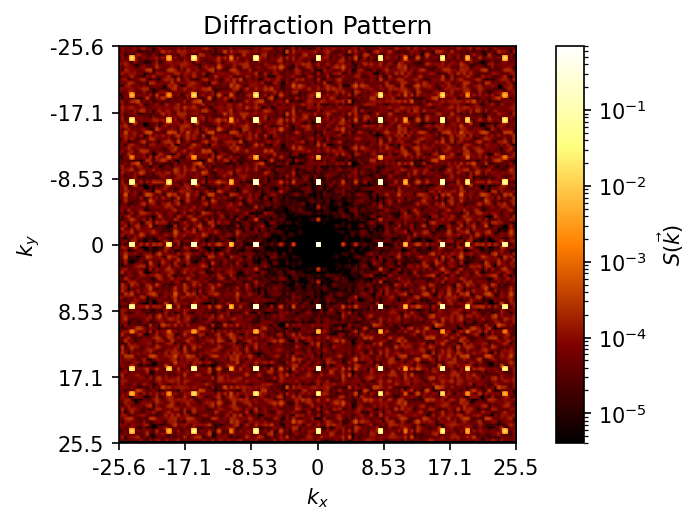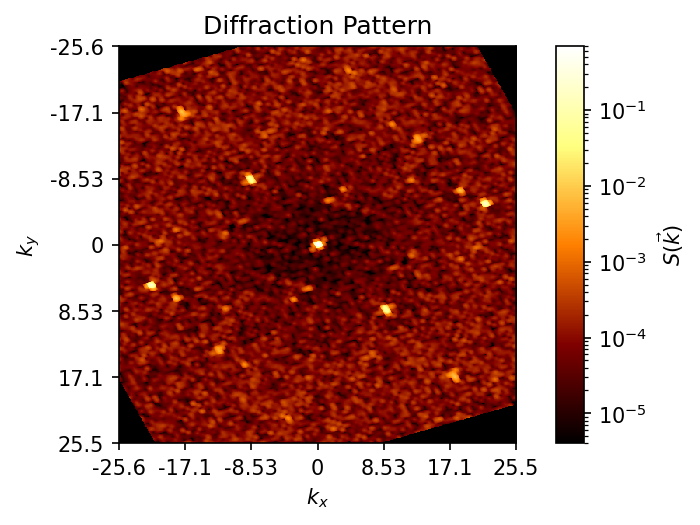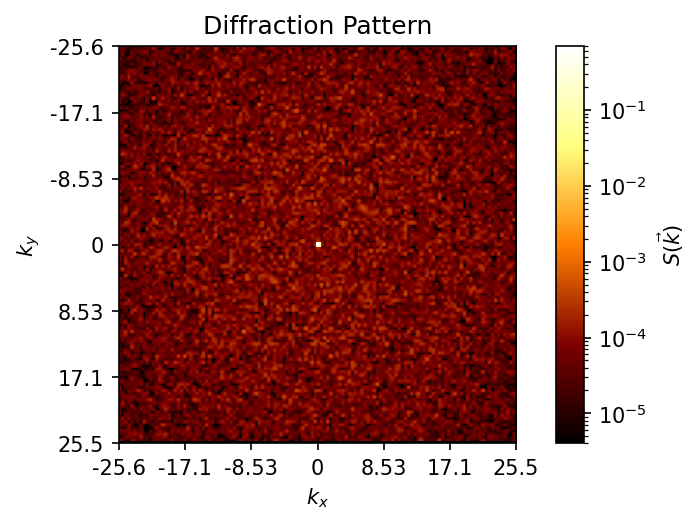# freud.diffraction.DiffractionPattern¶

The freud.diffraction.DiffractionPattern class computes a diffraction pattern, which is a 2D image of the static structure factor $$S(\vec{k})$$ of a set of points.

:

import freud
import matplotlib.pyplot as plt
import numpy as np
import rowan


First, we generate a sample system, a face-centered cubic crystal with some noise.

:

box, points = freud.data.UnitCell.fcc().generate_system(num_replicas=10, sigma_noise=0.02)


Now we create a DiffractionPattern compute object.

:

dp = freud.diffraction.DiffractionPattern(grid_size=512, output_size=512)


Next, we use the compute method and plot the result. We use a view orientation with the identity quaternion [1, 0, 0, 0] so the view is aligned down the z-axis.

:

fig, ax = plt.subplots(figsize=(4, 4), dpi=150)
dp.compute((box, points), view_orientation=[1, 0, 0, 0])
dp.plot(ax)
plt.show()We can also use a random quaternion for the view orientation to see what the diffraction looks like from another axis.

:

fig, ax = plt.subplots(figsize=(4, 4), dpi=150)
np.random.seed(0)
view_orientation = rowan.random.rand()
dp.compute((box, points), view_orientation=view_orientation)
print('Looking down the axis:', rowan.rotate(view_orientation, [0, 0, 1]))
dp.plot(ax)
plt.show()

Looking down the axis: [0.75707404 0.33639217 0.56007071]The DiffractionPattern object also provides $$\vec{k}$$ vectors in the original 3D space and the magnitudes of $$k_x$$ and $$k_y$$ in the 2D projection along the view axis.

:

print('Magnitudes of k_x and k_y along the plot axes:')
print(dp.k_values[:5], '...', dp.k_values[-5:])

Magnitudes of k_x and k_y along the plot axes:
[-25.6 -25.5 -25.4 -25.3 -25.2] ... [25.1 25.2 25.3 25.4 25.5]

:

print('3D k-vectors corresponding to each pixel of the diffraction image:')
print('Array shape:', dp.k_vectors.shape)
print('Center value: k =', dp.k_vectors[dp.output_size//2, dp.output_size//2, :])
print('Top-left value: k =', dp.k_vectors[0, 0, :])

3D k-vectors corresponding to each pixel of the diffraction image:
Array shape: (512, 512, 3)
Center value: k = [0. 0. 0.]
Top-left value: k = [ 19.03669015 -29.77826902  -7.84723661]


We can also measure the diffraction of a random system (note: this is an ideal gas, not a liquid-like system, because the particles have no volume exclusion or repulsion). Note that the peak at $$\vec{k} = 0$$ persists. The diffraction pattern returned by this class is normalized by dividing by $$N^2$$, so $$S(\vec{k}=0) = 1$$ after normalization.

:

box, points = freud.data.make_random_system(box_size=10, num_points=10000)
fig, ax = plt.subplots(figsize=(4, 4), dpi=150)
dp.compute((box, points))
dp.plot(ax)
plt.show()